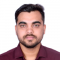## How to plot multiple horizontal bars in one chart with matplotlib?Rishikesh Kumar Rishi

Updated on 01-Feb-2022 11:14:19

To plot multiple horizontal bars in one chart with matplotlib, we can take the following steps −StepsImport the libraries pandas, matplotlib, and numpy.Set the figure size and adjust the padding between and around the subplots.Create an array for horizontal bar's position.Initialize a variable width for bar's width.Create a horizontal bar ... Read More

## How to shift the colorbar position to right in matplotlib?Rishikesh Kumar Rishi

Updated on 01-Feb-2022 11:11:11

To shift the colorbar position to right in matplotlib, we can take the following steps −StepsImport numpy and matplotlib.Set the figure size and adjust the padding between and around the subplots.Initialize a variable N to store the number of sample data.Create x and y data points using numpy.Create a scatter ... Read More

## How to set the value of the axis multiplier in matplotlib?Rishikesh Kumar Rishi

Updated on 01-Feb-2022 11:07:43

To set the value of the axis multiplier in matplotlib, we can take the following steps −StepsSet the figure size and adjust the padding between and around the subplots.Create x data points using numpy.Plot x and x2 using plot() method.Get the current axis of the figure.Initialize a variable multiplier, i.e., ... Read More

## In Matplotlib, show the percentage or proportional data where each slice of pie represents a categoryRishikesh Kumar Rishi

Updated on 26-Oct-2021 13:02:12

In this article, we can create a pie chart to show our daily activities, i.e., sleeping, eating, working, and playing. Using plt.pie() method, we can create a pie chart with the given different data sets for different activities.StepsCreate a list of days, i.e., [1, 2, 3, 4, 5]. Similarly, make ... Read More

## What is the equivalent of Matlab's surf(x,y,z,c) in Matplotlib?Rishikesh Kumar Rishi

Updated on 21-Oct-2021 08:48:15

Let's take an example to see how to get the same effect as MatLab's surf(x, y, z, c) in Matplotlib. steps −Set the figure size and adjust the padding between and around the subplots.Create a new figure or activate an existing figure.Add an 'ax' to the figure as part of ... Read More

## Animation with contours in matplotlibRishikesh Kumar Rishi

Updated on 19-Oct-2021 09:10:27

To animate with contours in matplotlib, we can take the following stepsStepsSet the figure size and adjust the padding between and around the subplots.Create data for the contour plot.Create a figure and a set of subplots.Generate an animation by repeatedly calling a function *animate* where the animate() method changes the ... Read More

## How to curve text in a polar plot in matplotlib?Rishikesh Kumar Rishi

Updated on 19-Oct-2021 08:41:45

To curve text in a polar plot in matplotlib we can take the following stepsStepsSet the figure size and adjust the padding between and around the subplots.Create a new figure or activate an existing figure.Add an 'ax' to the figure as part of a subplot arrangement.Plot the line with some ... Read More

## How get the (x,y) position pointing with mouse in an interactive plot (Python Matplotlib)?Rishikesh Kumar Rishi

Updated on 19-Oct-2021 08:36:26

To get the (x, y) positions pointing with mouse in an interactive plot, we can take the following stepsStepsSet the figure size and adjust the padding between and around the subplots.Create a new figure or activate an existing figure.Bind the function *mouse_event* to the event *button_press_event*.Create x and y data ... Read More

## How to put xtick labels in a box matplotlib?Rishikesh Kumar Rishi

Updated on 19-Oct-2021 08:29:23

To put xtick labels in a box, we can take the following stepsStepsCreate a new figure or activate an existing figure.Get the current axis of the figure.Set the left and bottom position of the axes.Set the position of the spines, i.e., bottom and left.To put xtick labels in a box, ... Read More

## How to plot a time as an index value in a Pandas dataframe in Matplotlib?Rishikesh Kumar Rishi

Updated on 19-Oct-2021 08:28:32

To plot a time as an index value in a Pandas dataframe in matplotlib, we can take the following stepsStepsSet the figure size and adjust the padding between and around the subplots.Create a Pandas dataframe with two columns, time and speed.Set the DataFrame index using existing columns.To display the figure, ... Read More

Previous 1 ... 3 4 5 6 7 ... 118 Next